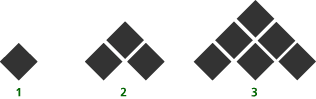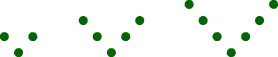# Classroom Case Studies, Grades 6-8 Part E: Critiquing Student Lessons (20 minutes)

## Session 10: 6-8, Part E

In the Grades 6-8 curriculum, students are frequently asked to think about patterns, but often their “pattern sniffing” skills end with simply finding the next object.

For each of the problems below, answer the following questions:
Note 8

 a. What algebraic ideas are in this problem? b. How are patterns used in this problem? c. What mathematics do you think students would learn from this problem? d. How would you modify the problem, or what additional questions might you ask, to incorporate the framework for analyzing patterns?

### Problems

Problem E1

How many tiles will be in the eighth figure of this pattern? How do you know?Problem E2a. Make a drawing of the next V-patterns. b. Is it possible for a V-pattern to have 84 dots? Why or why not? c. How many pairs are there in each V-pattern? d. How many dots will be in the sixth V-pattern? e. Make a V-pattern with 19 dots. f. Fill in the missing values in the table below. V-number means the number of pairs. Describe any patterns you see.
 V-Number Number of Dots1 3 2 5 3 7 4 ? 5 ? 6 ?

### Notes

Note 8

The two lessons in this section take a qualitatively different approach to students’ thinking about patterns. The types of questions asked in the Math in Context patterns problem allow students to use the physical representation to make conjectures about the pattern’s rule. In the first problem from Gateways, students are simply asked to supply a specific term in the sequence. In the framework developed on patterns, we were careful to include habits of mind that extend beyond finding a specific term. How do you know there are a specific number of dots or blocks in the sequence? If you know one term in the sequence, how could you get the next? Can you find a rule that will give you the number of dots or blocks for any term? These kinds of questions extend students’ thinking about predicting with patterns.

### Solutions

Problem E1

 a. This problem shows the first three figures of a growing pattern, and requires students to find the number of tiles needed to make the 8th figure in the pattern. b. This problem uses a growing pattern, and the nth term uses n more tiles than the previous term. c. This problem can be used to help students learn to write a general rule for the nth term of a function. d. This problem could be extended to find the number of tiles needed for the 10th, 100th, and then the nth term. Ask students to describe how to build each term. Look for answers that relate each term to the previous term, and then relate the figure to its place in the pattern. For example, students might notice that the 1st term uses 1 tile, the 2nd term uses 1 + 2, or 3 tiles, the 3rd term uses 1 + 2 + 3, or 6 tiles, and so forth. The nth term uses 1 + 2 + 3 + … + n tiles.

Problem E2

 a. This problem also shows the first three figures of a growing pattern. This problem not only requires students to find the number of dots needed to make the 6th figure in the pattern, however; it also requires students to determine whether it is possible for one of the terms to use 84 dots. b. This problem uses a growing pattern, and the nth term uses 2 more dots than the previous term. c. This problem can be used to help students learn to write a general rule for the nth term of a function. d. This problem asks students to find the number of dots needed for the 100th term, but could be further extended to ask for the number of dots needed for the nth term. Again ask students to describe how to build each term. Look for answers that relate each figure to its place in the pattern. For example, students might notice that the 1st term uses 1 dot + 1 pair of dots, the 2nd term uses 1 dot + 2 pairs of dots, the 3rd term uses 1 dot + 3 pairs of dots, and so forth. The nth term uses 1 dot + n pairs of dots, for a total of 2n + 1 dots.